xNon-equilibrium thermodynamicsEncyclopedia
Non-equilibrium thermodynamics is a branch of thermodynamics
Thermodynamics
Thermodynamics is a physical science that studies the effects on material bodies, and on radiation in regions of space, of transfer of heat and of work done on or by the bodies or radiation...

that deals with systems that are not in thermodynamic equilibrium
Thermodynamic equilibrium
In thermodynamics, a thermodynamic system is said to be in thermodynamic equilibrium when it is in thermal equilibrium, mechanical equilibrium, radiative equilibrium, and chemical equilibrium. The word equilibrium means a state of balance...

. Most systems found in nature are not in thermodynamic equilibrium; for they are changing or can be triggered to change over time, and are continuously and discontinuously subject to flux of matter and energy to and from other systems and to chemical reactions. Non-equilibrium thermodynamics is concerned with transport processes and with the rates of chemical reactions. Many natural systems still today remain beyond the scope of currently known macroscopic thermodynamic methods.

The thermodynamic study of non-equilibrium systems requires more general concepts than are dealt with by equilibrium thermodynamics
Equilibrium thermodynamics
Equilibrium Thermodynamics is the systematic study of transformations of matter and energy in systems as they approach equilibrium. The word equilibrium implies a state of balance. Equilibrium thermodynamics, in origins, derives from analysis of the Carnot cycle. Here, typically a system, as...

. One fundamental difference between equilibrium thermodynamics and non-equilibrium thermodynamics lies in the behaviour of inhomogeneous systems, which require for their study knowledge of rates of reaction which are not considered in equilibrium thermodynamics of homogeneous systems. This is discussed below. Another fundamental difference is the difficulty in defining entropy
Entropy
Entropy is a thermodynamic property that can be used to determine the energy available for useful work in a thermodynamic process, such as in energy conversion devices, engines, or machines. Such devices can only be driven by convertible energy, and have a theoretical maximum efficiency when...

in macroscopic terms for systems not in thermodynamic equilibrium.

## Overview

Non-equilibrium thermodynamics is a work in progress, not an established edifice. This article cannot give a full account of it. This article will try to sketch some approaches to it and some concepts important for it.

Some concepts of particular importance for non-equilibrium thermodynamics include time rate of dissipation of energy (Rayleigh 1873, Onsager 1931, also), time rate of entropy production (Onsager 1931), thermodynamic fields, dissipative structure, and non-linear dynamical structure.

Of interest is the thermodynamic study of non-equilibrium steady state
A system in a steady state has numerous properties that are unchanging in time. This implies that for any property p of the system, the partial derivative with respect to time is zero:...

s, in which entropy
Entropy
Entropy is a thermodynamic property that can be used to determine the energy available for useful work in a thermodynamic process, such as in energy conversion devices, engines, or machines. Such devices can only be driven by convertible energy, and have a theoretical maximum efficiency when...

production and some flows are non-zero, but there is no time variation
Time-variant system
A time-variant system is a system that is not time invariant . Roughly speaking, characteristics of its output depend explicitly upon time.- Overview :...

.

One initial approach to non-equilibrium thermodynamics might be called 'quasi-zero-dimensional'. There are other approaches to non-equilibrium thermodynamics, for example 'extended irreversible thermodynamics', but they are hardly touched on in the present article.

### Quasi-radiationless non-equilibrium thermodynamics of matter in laboratory conditions

According to Wildt (see also Essex), current versions of non-equilibrium thermodynamics ignore radiant heat; they can do so because they refer to laboratory quantities of matter under laboratory conditions with temperatures well below the those of stars. At laboratory temperatures, in laboratory quantities of matter, thermal radiation is weak and can be practically nearly ignored. For example, atmospheric physics is concerned with large amounts of matter, occupying cubic kilometers, that are not necessarily within the scope of laboratory conditions.

### 'Quasi-zero-dimensional' non-equilibrium thermodynamics

'Quasi-zero-dimensional' non-equilibrium thermodynamics demands certain simplifying assumptions, as follows. The assumptions have the effect of making the system effectively homogeneous, or well-mixed, or without an effective spatial structure, and without kinetic energy of bulk flow or diffusive flux. Even within the thought-frame of quasi-zero-dimensional non-equilibrium thermodynamics, care is needed in choosing the independent variables for systems. In some writings, it is assumed that the intensive variables of equilibrium thermodynamics are sufficient as the independent variables for the task (such variables are considered to have no 'memory', and do not show hysteresis); in particular, local flow intensive variables are not admitted as independent variables; local flows are considered as dependent on static local intensive variables. (In other writings, local flow variables are considered; examples of the quasi-zero-dimensional approach with flow are in the thermoelectric phenomena
Thermoelectric effect
The thermoelectric effect is the direct conversion of temperature differences to electric voltage and vice-versa. A thermoelectric device creates a voltage when there is a different temperature on each side. Conversely, when a voltage is applied to it, it creates a temperature difference...

known as the Seebeck and the Peltier effects, considered by Kelvin
William Thomson, 1st Baron Kelvin
William Thomson, 1st Baron Kelvin OM, GCVO, PC, PRS, PRSE, was a mathematical physicist and engineer. At the University of Glasgow he did important work in the mathematical analysis of electricity and formulation of the first and second laws of thermodynamics, and did much to unify the emerging...

in the nineteenth century and by Onsager
Lars Onsager
Lars Onsager was a Norwegian-born American physical chemist and theoretical physicist, winner of the 1968 Nobel Prize in Chemistry.He held the Gibbs Professorship of Theoretical Chemistry at Yale University....

in the twentieth. These effects occur at metal junctions, which were originally effectively treated as two-dimensional surfaces, with no spatial volume, and no spatial variation.) Also it is assumed that the local entropy density is the same function of the other local intensive variables as in equilibrium; this is called the local thermodynamic equilibrium assumption (see also Keizer (1987)). In the quasi-zero-dimensional approach, it is usually assumed that there is no bulk flow of ponderable matter, so that kinetic energy does not need to be considered. Radiation is also ignored because it is transfer of energy between regions, which can be remote from one another. In a slight extension of quasi-zero dimensional non-equilibrium thermodynamics, spatial variation is allowed but it is assumed that the global entropy of the system can be found by simple spatial integration of the local entropy density; this means that spatial structure cannot contribute as it properly should to the global entropy assessment for the system. Also assumed in this slight extension is spatial and temporal continuity and even differentiability of locally defined intensive variables such as temperature and internal energy density. All of these are very stringent demands. Consequently, this approach can deal with only a very limited range of phenomena. This approach is nevertheless valuable as an introduction to non-equilibrium thermodynamics.

## Basic concepts

There are many examples of stationary non-equilibrium systems, some very simple, like a system confined between two thermostats at different temperatures or the ordinary Couette flow
Couette flow
In fluid dynamics, Couette flow refers to the laminar flow of a viscous fluid in the space between two parallel plates, one of which is moving relative to the other. The flow is driven by virtue of viscous drag force acting on the fluid and the applied pressure gradient parallel to the plates...

, a fluid enclosed between two flat walls moving in opposite directions and defining non-equilibrium conditions at the walls. Laser
Laser
A laser is a device that emits light through a process of optical amplification based on the stimulated emission of photons. The term "laser" originated as an acronym for Light Amplification by Stimulated Emission of Radiation...

action is also a non-equilibrium process, but it depends on departure from local thermodynamic equilibrium and is thus beyond the scope of classical irreversible thermodynamics; here a strong temperature difference is maintained between two molecular degrees of freedom (with molecular laser, vibrational and rotational molecular motion), the requirement for two component 'temperatures' in the one small region of space, precluding local thermodynamic equilibrium, which demands that only one temperature be needed. Damping of acoustical perturbations or shock waves are non-stationary non-equilibrium processes. Driven complex fluids
Complex fluids
Complex fluids are binary mixtures that have a coexistence between two phases: solid–liquid , solid–gas , liquid–gas and liquid–liquid . They exhibit unusual mechanical responses to applied stress or strain due to the geometrical constraints that the phase coexistence imposes...

, turbulent systems and glasses are other examples of non-equilibrium systems.

The mechanics of macroscopic systems depends on a number of extensive quantities. It should be stressed that all systems are permanently interacting with their surroundings, thereby causing unavoidable fluctuations of extensive quantities. Equilibrium conditions of thermodynamic systems are related to the maximum property of the entropy. If the only extensive quantity that is allowed to fluctuate is the internal energy, all the other ones being kept strictly constant, the temperature of the system is measurable and meaningful. The system's properties are then most conveniently described using the thermodynamic potential Helmholtz free energy
Helmholtz free energy
In thermodynamics, the Helmholtz free energy is a thermodynamic potential that measures the “useful” work obtainable from a closed thermodynamic system at a constant temperature and volume...

(A = U - TS), a Legendre transformation
Legendre transformation
In mathematics, the Legendre transformation or Legendre transform, named after Adrien-Marie Legendre, is an operation that transforms one real-valued function of a real variable into another...

of the energy. If, next to fluctuations of the energy, the macroscopic dimensions (volume) of the system are left fluctuating, we use the Gibbs free energy
Gibbs free energy
In thermodynamics, the Gibbs free energy is a thermodynamic potential that measures the "useful" or process-initiating work obtainable from a thermodynamic system at a constant temperature and pressure...

(G = U + PV - TS), where the system's properties are determined both by the temperature and by the pressure.
Non-equilibrium systems are much more complex and they may undergo fluctuations of more extensive quantities. The boundary conditions impose on them particular intensive variables, like temperature gradients or distorted collective motions (shear motions, vortices, etc.), often called thermodynamic forces. If free energies are very useful in equilibrium thermodynamics, it must be stressed that there is no general law defining stationary non-equilibrium properties of the energy as is the second law of thermodynamics for the entropy
Entropy
Entropy is a thermodynamic property that can be used to determine the energy available for useful work in a thermodynamic process, such as in energy conversion devices, engines, or machines. Such devices can only be driven by convertible energy, and have a theoretical maximum efficiency when...

in equilibrium thermodynamics. That is why in such cases a more generalized Legendre transformation should be considered. This is the extended Massieu potential.
By definition, the entropy
Entropy
Entropy is a thermodynamic property that can be used to determine the energy available for useful work in a thermodynamic process, such as in energy conversion devices, engines, or machines. Such devices can only be driven by convertible energy, and have a theoretical maximum efficiency when...

(S) is a function of the collection of extensive quantities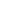. Each extensive quantity has a conjugate intensive variable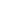(a restricted definition of intensive variable is used here by comparison to the definition given in this link) so that: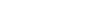We then define the extended Massieu function as follows: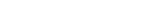where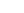is Boltzmann's constant, whence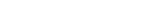The independent variables are the intensities.

Intensities are global values, valid for the system as a whole. When boundaries impose to the system different local conditions, (e.g. temperature differences), there are intensive variables representing the average value and others representing gradients or higher moments. The latter are the thermodynamic forces driving fluxes of extensive properties through the system.

It may be shown that the Legendre transformation changes the maximum condition of the entropy (valid at equilibrium) in a minimum condition of the extended Massieu function for stationary states, no matter whether at equilibrium or not.

## Stationary states, fluctuations, and stability

In thermodynamics one is often interested in a stationary state of a process, allowing that the stationary state include the occurrence of unpredictable and experimentally unreproducible fluctuations in the state of the system. The fluctuations are due to the system's internal sub-processes and to exchange of matter or energy with the system's surroundings that create the constraints that define the process.

If the stationary state of the process is stable, then the unreproducible fluctuations involve local transient decreases of entropy. The reproducible response of the system is then to increase the entropy back to its maximum by irreversible processes: the fluctuation cannot be reproduced with a significant level of probability. Fluctuations about stable stationary states are extremely small except near critical points (Kondepudi and Prigogine 1998, page 323). The stable stationary state has a local maximum of entropy and is locally the most reproducible state of the system. There are theorems about the irreversible dissipation of fluctuations. Here 'local' means local with respect to the abstract space of thermodynamic coordinates of state of the system.

If the stationary state is unstable, then any fluctuation will almost surely trigger the virtually explosive departure of the system from the unstable stationary state. This can be accompanied by increased export of entropy.

## Local thermodynamic equilibrium

The scope of present-day non-equilibrium thermodynamics does not cover all physical processes. A condition for the validity of many studies in non-equilibrium thermodynamics of matter is that they deal with what is known as local thermodynamic equilibrium.

### Local thermodynamic equilibrium of ponderable matter

Local thermodynamic equilibrium of matter (see also Keizer (1987) means that conceptually, for study and analysis, the system can be spatially and temporally divided into 'cells' or 'micro-phases' of small (infinitesimal) size, in which classical thermodynamical equilibrium conditions for matter are fulfilled to good approximation. These conditions are unfulfilled, for example, in very rarefied gases, in which molecular collisions are infrequent; and in the boundary layers of a star, where radiation is passing energy to space; and for interacting fermions at very low temperature, where dissipative processes become ineffective. When these 'cells' are defined, one admits that matter and energy may pass freely between contiguous 'cells', slowly enough to leave the 'cells' in their respective individual local thermodynamic equilibria with respect to intensive variables.

One can think here of two 'relaxation times' separated by order of magnitude. The longer relaxation time is of the order of magnitude of times taken for the macroscopic dynamical structure of the system to change. The shorter is of the order of magnitude of times taken for a single 'cell' to reach local thermodynamic equilibrium. If these two relaxation times are not well separated, then the classical non-equilibrium thermodynamical concept of local thermodynamic equilibrium loses its meaning. For example, in the atmosphere, the speed of sound is much greater than the wind speed; this favours the idea of local thermodynamic equilibrium of matter for atmospheric heat transfer studies at altitudes below about 60 km where sound propagates, but not above 100 km, where, because of the paucity of intermolecular collisions, sound does not propagate.

### Milne's 1928 definition of local thermodynamic equilibrium in terms of radiative equilibrium

Milne (1928), thinking about stars, gave a definition of 'local thermodynamic equilibrium' in terms of the thermal radiation
Thermal radiation is electromagnetic radiation generated by the thermal motion of charged particles in matter. All matter with a temperature greater than absolute zero emits thermal radiation....

of the matter
Matter
Matter is a general term for the substance of which all physical objects consist. Typically, matter includes atoms and other particles which have mass. A common way of defining matter is as anything that has mass and occupies volume...

in each small local 'cell'. He defined 'local thermodynamic equilibrium' in a 'cell' by requiring that it macroscopically absorb and spontaneously emit radiation as if it were in radiative equilibrium in a cavity at the temperature
Temperature
Temperature is a physical property of matter that quantitatively expresses the common notions of hot and cold. Objects of low temperature are cold, while various degrees of higher temperatures are referred to as warm or hot...

of the matter of the 'cell'. Then it strictly obeys Kirchhoff's law of equality of radiative emissivity and absorptivity, with a black body source function. The key to local thermodynamic equilibrium here is that the rate of collisions of ponderable matter particles such as molecules should far exceed the rates of creation and annihilation of photons.

## Entropy in evolving systems

It is pointed out by W.T. Grandy Jr that entropy, though it may be defined for a non-equilibrium system, is when strictly considered, only a macroscopic quantity that refers to the whole system, and is not a dynamical variable and in general does not act as a local potential that describes local physical forces. Under special circumstances, however, one can metaphorically think as if the thermal variables behaved like local physical forces. The approximation that constitutes classical irreversible thermodynamics is built on this metaphoric thinking.

## Flows and forces

The fundamental relation of classical equilibrium thermodynamics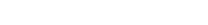expresses the change in entropy
Entropy
Entropy is a thermodynamic property that can be used to determine the energy available for useful work in a thermodynamic process, such as in energy conversion devices, engines, or machines. Such devices can only be driven by convertible energy, and have a theoretical maximum efficiency when...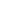of a system as a function of the intensive quantities temperature
Temperature
Temperature is a physical property of matter that quantitatively expresses the common notions of hot and cold. Objects of low temperature are cold, while various degrees of higher temperatures are referred to as warm or hot...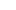, pressure
Pressure
Pressure is the force per unit area applied in a direction perpendicular to the surface of an object. Gauge pressure is the pressure relative to the local atmospheric or ambient pressure.- Definition :...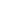and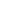chemical potential
Chemical potential
Chemical potential, symbolized by μ, is a measure first described by the American engineer, chemist and mathematical physicist Josiah Willard Gibbs. It is the potential that a substance has to produce in order to alter a system...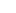and of the differentials of the extensive quantities energy
Energy
In physics, energy is an indirectly observed quantity. It is often understood as the ability a physical system has to do work on other physical systems...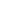, volume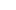andparticle number
Particle number
The particle number of a thermodynamic system, conventionally indicated with the letter N, is the number of constituent particles in that system. The particle number is a fundamental parameter in thermodynamics which is conjugate to the chemical potential. Unlike most physical quantities, particle...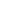.

Following Onsager (1931,I), let us extend our considerations to thermodynamically non-equilibrium systems. As a basis, we need locally defined versions of the extensive macroscopic quantities,andand of the intensive macroscopic quantities,and.

For classical non-equilibrium studies, we will consider some new locally defined intensive macroscopic variables. We can, under suitable conditions, derive these new variables by locally defining the gradients and flux densities of the basic locally defined macroscopic quantities.

Such locally defined gradients of intensive macroscopic variables are called 'thermodynamic forces'. They 'drive' flux densities, perhaps misleadingly often called 'fluxes', which are dual to the forces. These quantities are defined in the article on Onsager reciprocal relations
Onsager reciprocal relations
In thermodynamics, the Onsager reciprocal relations express the equality of certain ratios between flows and forces in thermodynamic systems out of equilibrium, but where a notion of local equilibrium exists....

.

Establishing the relation between such forces and flux densities is a problem in statistical mechanics. Flux densities (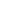) may be coupled. The article on Onsager reciprocal relations
Onsager reciprocal relations
In thermodynamics, the Onsager reciprocal relations express the equality of certain ratios between flows and forces in thermodynamic systems out of equilibrium, but where a notion of local equilibrium exists....

considers the stable near-steady thermodynamically non-equilibrium regime, which has dynamics linear in the forces and flux densities.

In stationary conditions, such forces and associated flux densities are by definition time invariant, as also are the system's locally defined entropy and rate of entropy production. Notably, according to Ilya Prigogine
Ilya Prigogine
Ilya, Viscount Prigogine was a Russian-born naturalized Belgian physical chemist and Nobel Laureate noted for his work on dissipative structures, complex systems, and irreversibility.-Biography :...

and others, when an open system is in conditions that allow it to reach a stable stationary thermodynamically non-equilibrium state, it organizes itself so as to minimize total entropy production defined locally. This is considered further below.

One wants to take the analysis to the further stage of describing the behaviour of surface and volume integrals of non-stationary local quantities; these integrals are macroscopic fluxes and production rates. In general the dynamics of these integrals are not adequately described by linear equations, though in special cases they can be so described.

## The Onsager relations

Following Section III of Rayleigh (1873), Onsager (1931, I) showed that in the regime where both the flows are small and the thermodynamic forces vary slowly, there will be a linear relation between them, parametrized by a matrix
Matrix (mathematics)
In mathematics, a matrix is a rectangular array of numbers, symbols, or expressions. The individual items in a matrix are called its elements or entries. An example of a matrix with six elements isMatrices of the same size can be added or subtracted element by element...

of coefficients conventionally denoted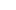: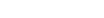The second law of thermodynamics
Second law of thermodynamics
The second law of thermodynamics is an expression of the tendency that over time, differences in temperature, pressure, and chemical potential equilibrate in an isolated physical system. From the state of thermodynamic equilibrium, the law deduced the principle of the increase of entropy and...

requires that the matrixbe positive definite. Statistical mechanics
Statistical mechanics
Statistical mechanics or statistical thermodynamicsThe terms statistical mechanics and statistical thermodynamics are used interchangeably...

considerations involving microscopic reversibility of dynamics imply that the matrixis symmetric. This fact is called the Onsager reciprocal relations.

## Speculated thermodynamic extremum principles for energy dissipation and entropy production

Jou, Casas-Vazquez, Lebon (1993) note that classical non-equilibrium thermodynamics "has seen an extraordinary expansion since the second world war", and they refer to the Nobel prizes for work in the field awarded to Lars Onsager
Lars Onsager
Lars Onsager was a Norwegian-born American physical chemist and theoretical physicist, winner of the 1968 Nobel Prize in Chemistry.He held the Gibbs Professorship of Theoretical Chemistry at Yale University....

and Ilya Prigogine
Ilya Prigogine
Ilya, Viscount Prigogine was a Russian-born naturalized Belgian physical chemist and Nobel Laureate noted for his work on dissipative structures, complex systems, and irreversibility.-Biography :...

. Martyushev and Seleznev (2006) note the importance of entropy in the evolution of natural dynamical structures: "Great contribution has been done in this respect by two scientists, namely Clausius, ... , and Prigogine
Ilya Prigogine
Ilya, Viscount Prigogine was a Russian-born naturalized Belgian physical chemist and Nobel Laureate noted for his work on dissipative structures, complex systems, and irreversibility.-Biography :...

." Prigogine in his 1977 Nobel Lecture said: "... non-equilibrium may be a source of order. Irreversible processes may lead to a new type of dynamic states of matter which I have called “dissipative structures”." Glansdorff and Prigogine (1971) wrote on page xx: "Such 'symmetry breaking instabilities' are of special interest as they lead to a spontaneous 'self-organization' of the system both from the point of view of its space order and its function."

Analyzing the Rayleigh-Bénard convection cell phenomenon, Chandrasekhar (1961) wrote "Instability occurs at the minimum temperature gradient at which a balance can be maintained between the kinetic energy dissipated by viscosity and the internal energy released by the buoyancy force." With a temperature gradient greater than the minimum, viscosity can dissipate kinetic energy as fast as it is released by convection due to buoyancy, and a steady state with convection is stable. The steady state with convection is often a pattern of macroscopically visible hexagonal cells with convection up or down in the middle or at the 'walls' of each cell, depending on the temperature dependence of the quantities; in the atmosphere under various conditions it seems that either is possible. (Some details are discussed by Lebon, Jou, and Casas-Vásquez (2008) on pages 143-158.) With a temperature gradient less than the minimum, viscosity and heat conduction are so effective that convection cannot keep going.

Glansdorff and Prigogine (1971) on page xv wrote "Dissipative structures have a quite different [from equilibrium structures] status: they are formed and maintained through the effect of exchange of energy and matter in non-equilibrium conditions." They were referring to the dissipation function of Rayleigh (1873) that was used also by Onsager (1931, I, 1931, II). On pages 78–80 of their book Glansdorff and Prigogine (1971) consider the stability of laminar flow that was pioneered by Helmholtz; they concluded that at a stable steady state of sufficiently slow laminar flow, the dissipation function was minimum.

These advances have led to proposals for various extremal principles
Extremal principles in non-equilibrium thermodynamics
Energy dissipation and entropy production extremal principles are ideas developed within non-equilibrium thermodynamics that attempt to predict the likely steady states and dynamical structures that a physical system might show. The search for extremum principles for non-equilibrium thermodynamics...

for the "self-organized
Self-organization
Self-organization is the process where a structure or pattern appears in a system without a central authority or external element imposing it through planning...

" régimes that are possible for systems governed by classical linear and non-linear non-equilibrium thermodynamical laws, with stable stationary régimes being particularly investigated. Convection introduces effects of momentum which appear as non-linearity in the dynamical equations. In the more restricted case of no convective motion, Prigogine wrote of "dissipative structures
Dissipative system
A dissipative system is a thermodynamically open system which is operating out of, and often far from, thermodynamic equilibrium in an environment with which it exchanges energy and matter....

". Šilhavý (1997) offers the opinion that "... the extremum principles of [equilibrium] thermodynamics ... do not have any counterpart for [non-equilibrium] steady states (despite many claims in the literature)."

### Prigogine’s proposed theorem of minimum entropy production

In 1945 Prigogine (see also Prigogine (1947)) proposed a “Theorem of Minimum Entropy Production” which applies only to the linear regime near a stationary thermodynamically non-equilibrium state. The proof offered by Prigogine is open to serious criticism. A critical and unsupportive discussion of Prigogine's proposal is offered by Grandy (2008).

### Speculated principles of maximum entropy production and minimum energy dissipation

Onsager (1931, I) wrote: "Thus the vector field J of the heat flow is described by the condition that the rate of increase of entropy, less the dissipation function, be a maximum." Careful note needs to be taken of the opposite signs of the rate of entropy production and of the dissipation function, appearing in the left-hand side of Onsager's equation (5.13) on Onsager's page 423.

Although largely unnoticed at the time, Ziegler proposed an idea early with his work in the mechanics of plastics in 1961, and later in his book on thermomechanics revised in 1983, and in various papers (e.g., Ziegler (1987),). Ziegler never stated his principle as a universal law but he may have intuited this. He demonstrated his principle using vector space geometry based on an “orthogonality condition” which only worked in systems where the velocities were defined as a single vector or tensor, and thus, as he wrote at p. 347, was “impossible to test by means of macroscopic mechanical models”, and was, as he pointed out, invalid in “compound systems where several elementary processes take place simultaneously”.

In relation to the earth's atmospheric energy transport process, according to Tuck (2008), "On the macroscopic level, the way has been pioneered by a meteorologist (Paltridge 1975, 2001)." Initially Paltridge (1975) used the terminology "minimum entropy exchange", but after that, for example in Paltridge (1978), and in Paltridge (1979), he used the now current terminology "maximum entropy production" to describe the same thing. The logic of Paltridge's work is open to serious criticism. Paltridge (1978) cited Busse's (1967) fluid mechanical work concerning an extremum principle, but it seems apparent that Paltridge was misinterpreting his source. Nicolis and Nicolis (1980) discuss Paltridge's work, and they comment that the behaviour of the entropy production is far from simple and universal.

Sawada (1981), also in relation to the earth's atmospheric energy transport process, postulating a principle of largest amount of entropy increment per unit time, cites work in fluid mechanics by Malkus and Veronis (1958) as having "proven a principle of maximum heat current, which in turn is a maximum entropy production for a given boundary condition", but this inference is not logically valid. Again investigating planetary atmospheric dynamics, Shutts (1981) used an approach to the definition of entropy production, different from Paltridge's, to investigate a more abstract way to check the principle of maximum entropy production, and reported a good fit.

### Prospects

At present, for this area of investigation, the prospects for useful extremal principles seem clouded at best. C. Nicolis (1999) concludes that one model of atmospheric dynamics has an attractor which is not a regime of maximum or minimum dissipation; she says this seems to rule out the existence of a global organizing principle, and comments that this is to some extent disappointing; she also points to the difficulty of finding a thermodynamically consistent form of entropy production; in the present writer's opinion, there are few as expert in the theory of entropy production as Nicolis. Another top expert offers an extensive discussion of the possibilities for principles of extrema of entropy production and of dissipation of energy: Chapter 12 of Grandy (2008) is very cautious, and finds difficulty in defining the 'rate of internal entropy production' in many cases, and finds that sometimes for the prediction of the course of a process, an extremum of the quantity called the rate of dissipation of energy may be more useful than that of the rate of entropy production; this quantity appeared in Onsager's 1931 origination of this subject.

These views on the difficulty or impossibility of finding general global extremal principles are consistent with the views of Glansdorff and Prigogine (1971), and of Lebon, Jou and Casas-Vásquez (2008), and of Šilhavý (1997), noted in the Wikipedia article on Extremal principles in non-equilibrium thermodynamics
Extremal principles in non-equilibrium thermodynamics
Energy dissipation and entropy production extremal principles are ideas developed within non-equilibrium thermodynamics that attempt to predict the likely steady states and dynamical structures that a physical system might show. The search for extremum principles for non-equilibrium thermodynamics...

, though more restricted local principles may exist.

## Applications of non-equilibrium thermodynamics

Non-equilibrium thermodynamics has been successfully applied to describe biological systems
such as Protein Folding/unfolding and transport through membranes.

• Dissipative system
Dissipative system
A dissipative system is a thermodynamically open system which is operating out of, and often far from, thermodynamic equilibrium in an environment with which it exchanges energy and matter....

• Extremal principles in non-equilibrium thermodynamics
Extremal principles in non-equilibrium thermodynamics
Energy dissipation and entropy production extremal principles are ideas developed within non-equilibrium thermodynamics that attempt to predict the likely steady states and dynamical structures that a physical system might show. The search for extremum principles for non-equilibrium thermodynamics...

• Self-organization
Self-organization
Self-organization is the process where a structure or pattern appears in a system without a central authority or external element imposing it through planning...

• Autocatalytic reactions and order creation
• Self-organizing criticality
• Bogoliubov-Born-Green-Kirkwood-Yvon hierarchy of equations
BBGKY hierarchy
In statistical physics, the BBGKY hierarchy is a set of equations describing the dynamics of a system of a large number of interacting particles...

• Boltzmann equation
Boltzmann equation
The Boltzmann equation, also often known as the Boltzmann transport equation, devised by Ludwig Boltzmann, describes the statistical distribution of one particle in rarefied gas...

• Vlasov equation
Vlasov equation
The Vlasov equation is a differential equation describing time evolution of the distribution function of plasma consisting of charged particles with long-range interaction...

• Maxwell's daemon
• Information entropy
Information entropy
In information theory, entropy is a measure of the uncertainty associated with a random variable. In this context, the term usually refers to the Shannon entropy, which quantifies the expected value of the information contained in a message, usually in units such as bits...

• Constructal theory
Constructal theory
The constructal law puts forth the idea that the generation of design in nature is a physics phenomenon that unites all animate and inanimate systems, and that this phenomenon is covered by the Constructal Law...

• Ziegler, Hans (1977): An introduction to Thermomechanics. North Holland, Amsterdam. ISBN 0444110801. Second edition (1983) ISBN 0444865039.
• Kleidon, A., Lorenz, R.D., editors (2005). Non-equilibrium Thermodynamics and the Production of Entropy, Springer, Berlin. ISBN 3540224955.
• Prigogine, I. (1955/1961/1967). Introduction to Thermodynamics of Irreversible Processes. 3rd edition, Wiley Interscience, New York.
• Zubarev D. N.
Dmitry Zubarev
Dmitry Nikolaevich Zubarev was a Russian theoretical physicist known for his contributions to statistical mechanics, non-equilibrium thermodynamics, plasma physics, theory of turbulence, and to the development of the double-time Green function's formalism....

(1974): Nonequilibrium Statistical Thermodynamics. New York, Consultants Bureau. ISBN 030610895X; ISBN 9780306108952.
• Keizer, J. (1987). Statistical Thermodynamics of Nonequilibrium Processes, Springer-Verlag, New York, ISBN 0387965017.
• Zubarev D. N.
Dmitry Zubarev
Dmitry Nikolaevich Zubarev was a Russian theoretical physicist known for his contributions to statistical mechanics, non-equilibrium thermodynamics, plasma physics, theory of turbulence, and to the development of the double-time Green function's formalism....

, Morozov V., Ropke G. (1996): Statistical Mechanics of Nonequilibrium Processes: Basic Concepts, Kinetic Theory. John Wiley & Sons. ISBN 3055017080.
• Zubarev D. N.
Dmitry Zubarev
Dmitry Nikolaevich Zubarev was a Russian theoretical physicist known for his contributions to statistical mechanics, non-equilibrium thermodynamics, plasma physics, theory of turbulence, and to the development of the double-time Green function's formalism....

, Morozov V., Ropke G. (1997): Statistical Mechanics of Nonequilibrium Processes: Relaxation and Hydrodynamic Processes. John Wiley & Sons. ISBN 3527400842.
• Tuck, Adrian F. (2008). Atmospheric turbulence : a molecular dynamics perspective. Oxford University Press. ISBN 9780199236534.
• Grandy, W.T., Jr (2008). Entropy and the Time Evolution of Macroscopic Systems. Oxford University Press. ISBN 9780199546176.
• Kondepudi, D., Prigogine, I. (1998). Modern Thermodynamics: From Heat Engines to Dissipative Structures. John Wiley & Sons, Chichester. ISBN 0471973939.